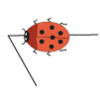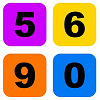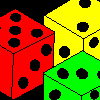Search by Topic

Resources tagged with Mathematical reasoning & proof similar to Sz'kwa:

Filter by: Content type:
Age range:
Challenge level:

There are 27 results

Broad Topics > Using, Applying and Reasoning about Mathematics > Mathematical reasoning & proofAge 5 to 11 Challenge Level:

Who said that adding couldn't be fun?Online

Age 7 to 11 Challenge Level:

A game for 2 players that can be played online. Players take it in turns to select a word from the 9 words given. The aim is to select all the occurrences of the same letter.A Bag of Marbles

Age 5 to 7 Challenge Level:

Use the information to describe these marbles. What colours must be on marbles that sparkle when rolling but are dark inside?Always, Sometimes or Never? Shape

Age 7 to 11 Challenge Level:

Are these statements always true, sometimes true or never true?Always, Sometimes or Never?

Age 5 to 11 Challenge Level:

Are these statements relating to odd and even numbers always true, sometimes true or never true?Take One Example

Age 5 to 11

This article introduces the idea of generic proof for younger children and illustrates how one example can offer a proof of a general result through unpacking its underlying structure.Problem Solving, Using and Applying and Functional Mathematics

Age 5 to 18 Challenge Level:

Problem solving is at the heart of the NRICH site. All the problems give learners opportunities to learn, develop or use mathematical concepts and skills. Read here for more information.Two Numbers Under the Microscope

Age 5 to 7 Challenge Level:

This investigates one particular property of number by looking closely at an example of adding two odd numbers together.Next-door Numbers

Age 5 to 7 Challenge Level:

Florence, Ethan and Alma have each added together two 'next-door' numbers. What is the same about their answers?Walking Round a Triangle

Age 5 to 7 Challenge Level:

This ladybird is taking a walk round a triangle. Can you see how much he has turned when he gets back to where he started?Square Subtraction

Age 7 to 11 Challenge Level:

Look at what happens when you take a number, square it and subtract your answer. What kind of number do you get? Can you prove it?Odd Times Even

Age 5 to 7 Challenge Level:

This problem looks at how one example of your choice can show something about the general structure of multiplication.Take Three Numbers

Age 7 to 11 Challenge Level:

What happens when you add three numbers together? Will your answer be odd or even? How do you know?Age 7 to 14 Challenge Level:

I added together some of my neighbours' house numbers. Can you explain the patterns I noticed?Air Nets

Age 7 to 18 Challenge Level:

Can you visualise whether these nets fold up into 3D shapes? Watch the videos each time to see if you were correct.Primary Proof?

Age 5 to 7

Proof does have a place in Primary mathematics classrooms, we just need to be clear about what we mean by proof at this level.Sprouts Explained

Age 7 to 18

This article invites you to get familiar with a strategic game called "sprouts". The game is simple enough for younger children to understand, and has also provided experienced mathematicians with. . . .What Do You Need?

Age 7 to 11 Challenge Level:

Four of these clues are needed to find the chosen number on this grid and four are true but do nothing to help in finding the number. Can you sort out the clues and find the number?Cows and Sheep

Age 7 to 11 Challenge Level:

Use your logical reasoning to work out how many cows and how many sheep there are in each field.Making Pathways

Age 7 to 11 Challenge Level:

Can you find different ways of creating paths using these paving slabs?Three Neighbours

Age 7 to 11 Challenge Level:

Look at three 'next door neighbours' amongst the counting numbers. Add them together. What do you notice?Always, Sometimes or Never? KS1

Age 5 to 7 Challenge Level:

Are these statements relating to calculation and properties of shapes always true, sometimes true or never true?Logic

Age 7 to 14

What does logic mean to us and is that different to mathematical logic? We will explore these questions in this article.Always, Sometimes or Never? Number

Age 7 to 11 Challenge Level:

Are these statements always true, sometimes true or never true?Age 7 to 14

A paradox is a statement that seems to be both untrue and true at the same time. This article looks at a few examples and challenges you to investigate them for yourself.